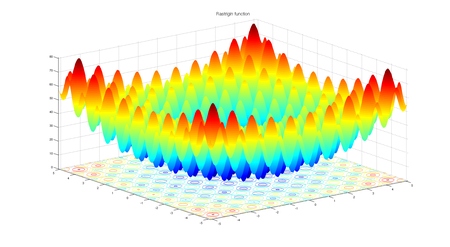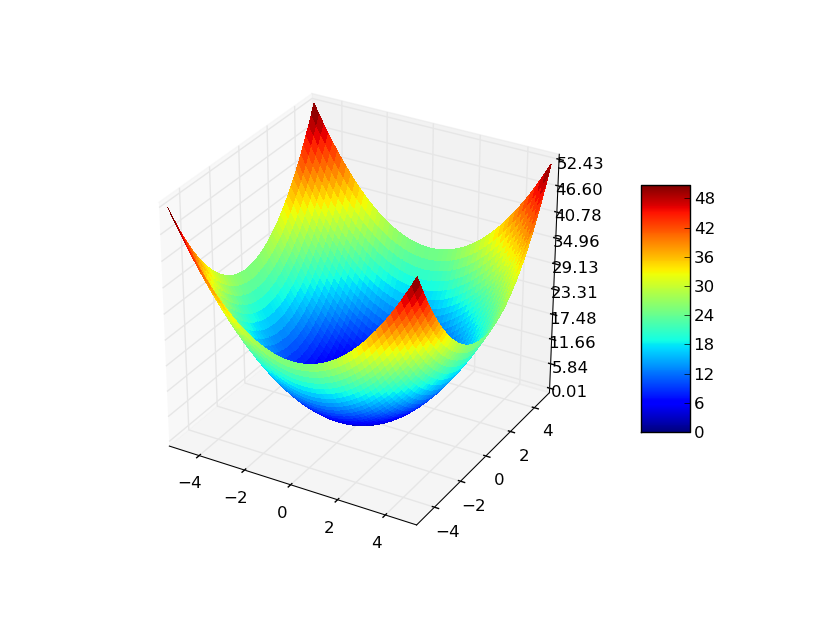Open in App
Not now

# Implementation of Particle Swarm Optimization

• Last Updated : 31 Aug, 2021

Previous article Particle Swarm Optimization – An Overview talked about inspiration of particle swarm optimization (PSO) , it’s mathematical modelling and algorithm. In this article we will implement particle swarm optimization (PSO) for two fitness functions 1) Rastrigin function    2) Sphere function.  The algorithm will run for a predefined number of maximum iterations and will try to find the minimum value of these fitness functions.

## Fitness functions

### 1) Rastrigin function

Rastrigin function is a non-convex function and is often used as a performance test problem for optimization algorithms.

#### function equation:Fig1: Rastrigin function for 2 variables

For an optimization algorithm, rastrigin function is a very challenging one. Its complex behavior cause optimization algorithms to often stuck at local minima. Having a lot of cosine oscillations on the plane introduces the complex behavior to this function.

### 2) Sphere function

Sphere function is a standard function for evaluating the performance of an optimization algorithm.

#### function equation:Fig2: Sphere function for 2 variables

## Choice of hyper-parameters

### Parameters of problem:

• Number of dimensions (d) = 3
• Lower bound (minx) = -10.0
• Upper bound (maxx) = 10.0

### Hyperparameters of the algorithm:

• Number of particles (N) = 50
• Maximum number of iterations (max_iter) = 100
• inertia coefficient (w) = 0.729
• cognitive coefficient (c1) = 1.49445
• social coefficient (c2) = 1.49445

### Inputs

• Fitness function
• Problem parameters ( mentioned above)
• Population size (N) and Maximum number of iterations  (max_iter)
• Algorithm Specific hyper parameters ( w, c1, c2)

## Pseudocode

The pseudocode of the particle swarm optimization is already described in the previous article. Data structures to store Swarm population, as well as a data structure to store data specific to individual particle, were also discussed.

## Python3

 # python implementation of particle swarm optimization (PSO)# minimizing rastrigin and sphere function import randomimport math    # cos() for Rastriginimport copy    # array-copying convenienceimport sys     # max float  #-------fitness functions--------- # rastrigin functiondef fitness_rastrigin(position):  fitnessVal = 0.0  for i in range(len(position)):    xi = position[i]    fitnessVal += (xi * xi) - (10 * math.cos(2 * math.pi * xi)) + 10  return fitnessVal #sphere functiondef fitness_sphere(position):    fitnessVal = 0.0    for i in range(len(position)):        xi = position[i]        fitnessVal += (xi*xi);    return fitnessVal;#------------------------- #particle classclass Particle:  def __init__(self, fitness, dim, minx, maxx, seed):    self.rnd = random.Random(seed)     # initialize position of the particle with 0.0 value    self.position = [0.0 for i in range(dim)]      # initialize velocity of the particle with 0.0 value    self.velocity = [0.0 for i in range(dim)]     # initialize best particle position of the particle with 0.0 value    self.best_part_pos = [0.0 for i in range(dim)]     # loop dim times to calculate random position and velocity    # range of position and velocity is [minx, max]    for i in range(dim):      self.position[i] = ((maxx - minx) *        self.rnd.random() + minx)      self.velocity[i] = ((maxx - minx) *        self.rnd.random() + minx)     # compute fitness of particle    self.fitness = fitness(self.position) # curr fitness     # initialize best position and fitness of this particle    self.best_part_pos = copy.copy(self.position)    self.best_part_fitnessVal = self.fitness # best fitness # particle swarm optimization functiondef pso(fitness, max_iter, n, dim, minx, maxx):  # hyper parameters  w = 0.729    # inertia  c1 = 1.49445 # cognitive (particle)  c2 = 1.49445 # social (swarm)   rnd = random.Random(0)   # create n random particles  swarm = [Particle(fitness, dim, minx, maxx, i) for i in range(n)]   # compute the value of best_position and best_fitness in swarm  best_swarm_pos = [0.0 for i in range(dim)]  best_swarm_fitnessVal = sys.float_info.max # swarm best   # computer best particle of swarm and it's fitness  for i in range(n): # check each particle    if swarm[i].fitness < best_swarm_fitnessVal:      best_swarm_fitnessVal = swarm[i].fitness      best_swarm_pos = copy.copy(swarm[i].position)   # main loop of pso  Iter = 0  while Iter < max_iter:         # after every 10 iterations    # print iteration number and best fitness value so far    if Iter % 10 == 0 and Iter > 1:      print("Iter = " + str(Iter) + " best fitness = %.3f" % best_swarm_fitnessVal)     for i in range(n): # process each particle             # compute new velocity of curr particle      for k in range(dim):        r1 = rnd.random()    # randomizations        r2 = rnd.random()             swarm[i].velocity[k] = (                                 (w * swarm[i].velocity[k]) +                                 (c1 * r1 * (swarm[i].best_part_pos[k] - swarm[i].position[k])) +                                  (c2 * r2 * (best_swarm_pos[k] -swarm[i].position[k]))                               )           # if velocity[k] is not in [minx, max]        # then clip it        if swarm[i].velocity[k] < minx:          swarm[i].velocity[k] = minx        elif swarm[i].velocity[k] > maxx:          swarm[i].velocity[k] = maxx        # compute new position using new velocity      for k in range(dim):        swarm[i].position[k] += swarm[i].velocity[k]         # compute fitness of new position      swarm[i].fitness = fitness(swarm[i].position)       # is new position a new best for the particle?      if swarm[i].fitness < swarm[i].best_part_fitnessVal:        swarm[i].best_part_fitnessVal = swarm[i].fitness        swarm[i].best_part_pos = copy.copy(swarm[i].position)       # is new position a new best overall?      if swarm[i].fitness < best_swarm_fitnessVal:        best_swarm_fitnessVal = swarm[i].fitness        best_swarm_pos = copy.copy(swarm[i].position)         # for-each particle    Iter += 1  #end_while  return best_swarm_pos# end pso  #----------------------------# Driver code for rastrigin function print("\nBegin particle swarm optimization on rastrigin function\n")dim = 3fitness = fitness_rastrigin  print("Goal is to minimize Rastrigin's function in " + str(dim) + " variables")print("Function has known min = 0.0 at (", end="")for i in range(dim-1):  print("0, ", end="")print("0)") num_particles = 50max_iter = 100 print("Setting num_particles = " + str(num_particles))print("Setting max_iter    = " + str(max_iter))print("\nStarting PSO algorithm\n")   best_position = pso(fitness, max_iter, num_particles, dim, -10.0, 10.0) print("\nPSO completed\n")print("\nBest solution found:")print(["%.6f"%best_position[k] for k in range(dim)])fitnessVal = fitness(best_position)print("fitness of best solution = %.6f" % fitnessVal) print("\nEnd particle swarm for rastrigin function\n")  print()print()  # Driver code for Sphere functionprint("\nBegin particle swarm optimization on sphere function\n")dim = 3fitness = fitness_sphere  print("Goal is to minimize sphere function in " + str(dim) + " variables")print("Function has known min = 0.0 at (", end="")for i in range(dim-1):  print("0, ", end="")print("0)") num_particles = 50max_iter = 100 print("Setting num_particles = " + str(num_particles))print("Setting max_iter    = " + str(max_iter))print("\nStarting PSO algorithm\n")   best_position = pso(fitness, max_iter, num_particles, dim, -10.0, 10.0) print("\nPSO completed\n")print("\nBest solution found:")print(["%.6f"%best_position[k] for k in range(dim)])fitnessVal = fitness(best_position)print("fitness of best solution = %.6f" % fitnessVal) print("\nEnd particle swarm for sphere function\n")

## Output:

Begin particle swarm optimization on rastrigin function

Goal is to minimize Rastrigin's function in 3 variables
Function has known min = 0.0 at (0, 0, 0)
Setting num_particles = 50
Setting max_iter    = 100

Starting PSO algorithm

Iter = 10 best fitness = 8.463
Iter = 20 best fitness = 4.792
Iter = 30 best fitness = 2.223
Iter = 40 best fitness = 0.251
Iter = 50 best fitness = 0.251
Iter = 60 best fitness = 0.061
Iter = 70 best fitness = 0.007
Iter = 80 best fitness = 0.005
Iter = 90 best fitness = 0.000

PSO completed

Best solution found:
['0.000618', '0.000013', '0.000616']
fitness of best solution = 0.000151

End particle swarm for rastrigin function

Begin particle swarm optimization on sphere function

Goal is to minimize sphere function in 3 variables
Function has known min = 0.0 at (0, 0, 0)
Setting num_particles = 50
Setting max_iter    = 100

Starting PSO algorithm

Iter = 10 best fitness = 0.189
Iter = 20 best fitness = 0.012
Iter = 30 best fitness = 0.001
Iter = 40 best fitness = 0.000
Iter = 50 best fitness = 0.000
Iter = 60 best fitness = 0.000
Iter = 70 best fitness = 0.000
Iter = 80 best fitness = 0.000
Iter = 90 best fitness = 0.000

PSO completed

Best solution found:
['0.000004', '-0.000001', '0.000007']
fitness of best solution = 0.000000

End particle swarm for sphere function

#### References

Research paper citation: Kennedy, J. and Eberhart, R., 1995, November. Particle swarm optimization. In Proceedings of ICNN’95-international conference on neural networks (Vol. 4, pp. 1942-1948). IEEE.

Inspiration of the implementation: https://fr.mathworks.com/matlabcentral/fileexchange/67429-a-simple-implementation-of-particle-swarm-optimization-pso-algorithm

My Personal Notes arrow_drop_up